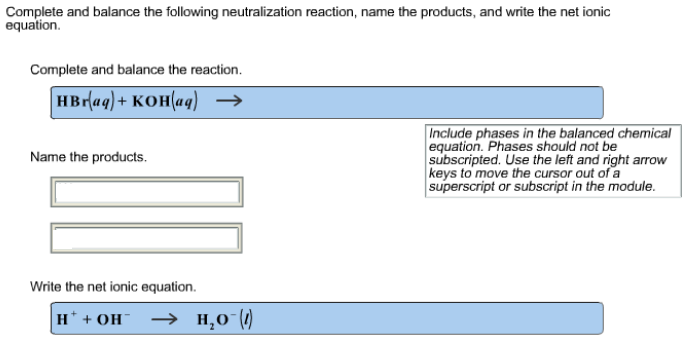# Problem: Complete and balance the following neutralization reaction, name the products, and write the net ionic equation. Complete and balance the reaction. HBr(aq) + KOH(aq) → Name the products. Write the net ionic equation. H+ + OH- → H2O-(l)

###### FREE Expert Solution
83% (496 ratings)###### Problem Details

Complete and balance the following neutralization reaction, name the products, and write the net ionic equation. Complete and balance the reaction.

HBr(aq) + KOH(aq) →

Name the products.

Write the net ionic equation.

H+ + OH- → H2O-(l)Frequently Asked Questions

What scientific concept do you need to know in order to solve this problem?

Our tutors have indicated that to solve this problem you will need to apply the Complete Ionic Equations concept. You can view video lessons to learn Complete Ionic Equations. Or if you need more Complete Ionic Equations practice, you can also practice Complete Ionic Equations practice problems.

What professor is this problem relevant for?

Based on our data, we think this problem is relevant for Professor Lamont's class at UW-MADISON.